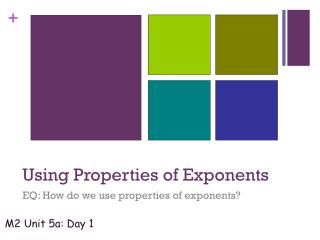DownloadDownload PresentationUsing Properties of Exponents

# Using Properties of Exponents

Download Presentation## Using Properties of Exponents

- - - - - - - - - - - - - - - - - - - - - - - - - - - E N D - - - - - - - - - - - - - - - - - - - - - - - - - - -
##### Presentation Transcript

1. Using Properties of Exponents EQ: How do we use properties of exponents? Monday, July 14, 2014 M2 Unit 5a: Day 1

2. Properties of Exponents Let a and b be real numbers and let m and n be integers. Product of Powers Property Power of a Power Property Power of a Product Property Zero Exponent Property Quotient of Powers Property Power of Quotient Property Negative Exponent Property

3. Product of Powers: To multiply powers with the same base, add the exponents and keep the common base. • Examples: a. b.

4. Your turn: 1. 2. 3. Keep common bases Add the exponents

5. Power of a Power: To raise a power to a power, keep the base and multiply the exponents. • Examples: a. b.

6. Your turn: 1. 2. 3. Keep The Base Multiply The Exponents

7. Power of a Product: To raise a product to a power, raise each factor to the power. • Examples: a. b. Simplify any numeric bases raised to exponents This should remind you of the distributive property. Remember to “distribute” the exponent to each base, numeric and variable! Warning: No distributing over addition!!!

8. Your turn: 1. 2. 3. Raise Each Factor To The Power It’s Like The Distributive Property

9. Zero Exponent: Any number raised to the zero power is equal to “1”. • Examples: a. b.

10. Your turn: 1. 2. 3. Anything Raised To The Zero Power =1

11. Quotient of Powers: To divide powers with the same base, subtract the exponents and keep the common base. Also, remember that common factors cancel out! • Examples: a. b. Keep the base where the exponent is larger

12. Your turn: 1. 2. 3. Common Factors Cancel Out With Common Bases Subtract Exponents

13. Power of Quotient: To raise a quotient to a power, raise the numerator and the denominator to the power. • Examples: a. b.

14. Raise the numerator and denominator to the power Your turn: 1. 2. 3. Simplify any factors with numeric bases

15. Negative Exponent Property: • You may want to think of it this way: unhappy ( ) exponents will become happy ( ) by having the base/exponent pair “switch floors”! • Example: A.) B.) positive negative

16. You try: 1. 2. 3. 4.

17. Simplifying Exponential Expressions To simplify exponential expressions completely: Get rid of parentheses Get rid of negative exponents Make sure there is only one of each base Cancel out common factors

18. Simplify the exponential expression. multistep C. Examples: A. B. D.

19. You try: multistep 1. 2. 3. 4.

20. A. B. C. D. multistep Simplify the exponential expression.

21. You Try:5.6. 7. multistep